The Precision Farming Primer      Topic 2: Point Sampling © 1999 Precision Farming Primer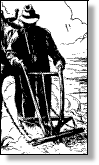presents an overview of the sampling process discusses sampling patterns and their implications describes important factors common in spatial interpolation discusses procedures and results of IDW, Kriging and MinCurve interpolation describes the Residual Analysis procedure for assessing interpolation results identifies a procedure for generating maps of error from Residual Analysis introduces the important concept of spatial dependency describes the information in a variogram plot of spatial dependency discusses fundamental differences between Management Zones and Map Surfaces

______________________________

Dirty Stuff

In topic 1. "Four Basic Steps in Precision Farming," the four basic processing steps in precision farming are briefly outlined:

• continuous data logging,
• point sampling,
• mapped data analysis and
• spatial modeling.

Now it’s time to forge ahead into the surrealistic realm of point sampling (i.e., soil mapping).  While data logging continuously records measurements (e.g., crop yield) as a tractor moves through a field, point sampling uses a set of dispersed, discrete samples to characterize field conditions (e.g., phosphorous).  The nature of the data derived by the two approaches is radically different—a "direct census" for a yield map versus a "statistical estimate" for a phosphorous map.  In data logging, issues of accurate measurement, such as GPS positioning and material flow adjustments, are major concerns.

In point sampling, issues of spatial interpolation—estimating between sample points such as sampling frequency/pattern and interpolation technique—are the focus of concern.

Let’s tackle the first area: the sampling design issues of frequency and pattern.   There are four distinct considerations in sampling design:

• stratification,
• sample size,
• sampling grid and
• sampling pattern.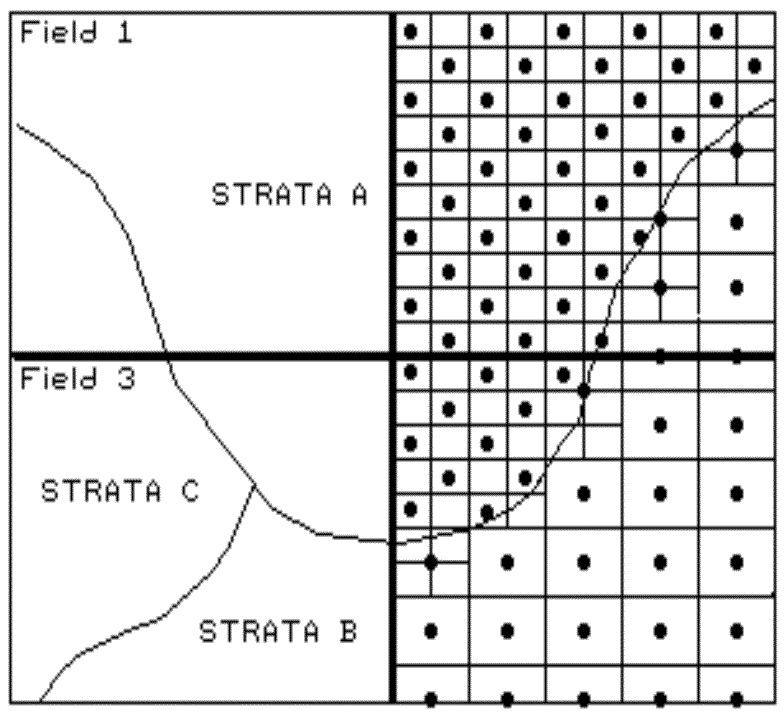Fig. 2.1. Field stratification.

The first three considerations determine the appropriate groupings for sampling (stratification), the sampling intensity for each group (sample size) and an appropriate reference grid resolution (sampling grid).  All are closely tied to the spatial variation of the data to be mapped.  For example, if your field splits a couple of soil types, you might divide the field into two strata.  If one is fairly consistent, you might allocate fewer samples than within the other, more variable soil unit (see fig. 2.1).  Also, you might decide to generate a third strata for even more intensive sampling around the soil boundary itself.  Or, another stratification possibility is to use your yield map.  If you feel the variations in yield are primarily "driven" by soil conditions, then that map could be used to divide the field into high, medium and low productivity areas.

This approach might respond to soil units that are below the resolution of the traditional soil type map or characterized by different nutrient levels.  In the past, a single sampling frequency was used throughout an entire state or region.  In part, a single frequency was chosen for ease and consistency of field implementation, as well as tradition.  With the advent of GPS technology, however, variable sample frequencies is a modern alternative¾ simply navigate to each sample location.

The final step, sampling pattern, identifies individual sample locations.   Traditional statistics tends to emphasize randomized patterns because they ensure maximum independence among samples, which is critical to calculating the central tendency of a data set (average for an entire field).  However, "the random thing" can actually hinder spatial statistic’s ability to map field variability.  Arguments supporting such statistical heresy involves the discussion of spatial dependency and autocorrelation, which mercifully is postponed until topic 2, "What's It Like Around Here," topic 3, "Assessing Spatial Dependency," and appendix A, part 2, "More on Assessing Spatial Dependency."  For the current discussion, let’s assume sampling patterns other than random are our best candidates.

Figure 2.2 identifies five systematic patterns, including a completely random one.   Note that the regular pattern is uniformly distributed in geographic space.   So is the staggered start one, except the equally spaced y-axis samples alternate the starting position at one-half the sampling grid spacing. The result is a "diamond" pattern rather than a "rectangular" one.  This pattern is better suited for generating maps because it provides more intersample distances for spatial interpolation.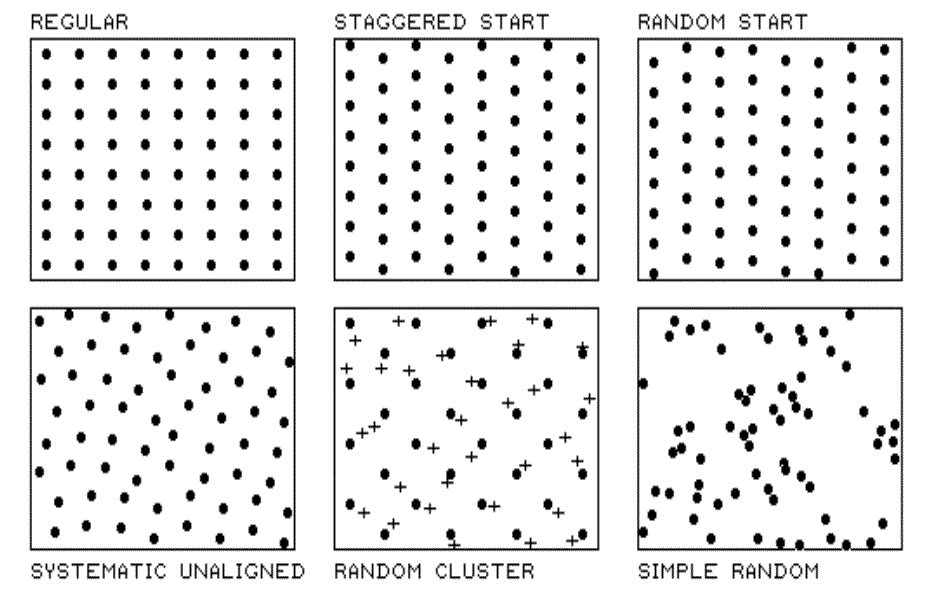Fig. 2.2. Systematic sampling patterns.

The random-start pattern begins each column transect at a randomly chosen y coordinate within the first grid cell, thereby creating even more intersample distances.   The result is nearly a regularly spaced pattern, with "just a hint of randomness." The systematic unaligned pattern also results in a fairly regularly spaced pattern, but it exhibits even more randomness as it is not aligned in either the x or y direction.  The pattern is formed by first placing a random point in the cell in the lower-left corner to establish a pair of x and y offsets.   For rows in the grid, the x offset is held constant while y is randomly varied. For columns, the y offset is held constant while x is varied.  The result is random starts in both the x and y axes.

The "dots" in the random cluster pattern establish an underlying uniform pattern (every other staggered start sample point).  The "crosses" locate a set of related samples that are randomly chosen within the enlarged grid space surrounding each dot.  Note that the patterned is not as regularly spaced as the previous techniques; however, it has other advantages.  The random subset of points allows a degree of unbiased statistical inference, such as a t-test of significance differences among population means.  The simple random pattern allows full use of statistical inference (whole field statistics), but the "clumping" of the samples makes it the worst for mapping (site-specific statistics).  So which pattern should be used? Whichever works best with your data and objectives.

Spatial interpolation refers to the generation of a continuous map surface from discrete point samples.  All spatial interpolation techniques establish a "roving window" that

• moves to a location in a field,
• calculates an estimate (guess) based on the point samples around it,
• assigns the estimate to the center of the window and
• moves to the next location.

The extent of the window (both size and shape) affects the result, regardless of the summary technique.  In general, a large window capturing a bunch of values tends to "smooth" the data.  A smaller window tends to result in a "rougher" surface with abrupt transitions.

Three factors affect the window's extent: its reach, the number of samples, balancing.   The reach, or search radius, sets a limit on how far the computer will go in collecting data values.  The number of samples establishes how many data values should be used.  If there are more than enough values within a specified reach, the computer uses just the closest ones.  If there aren't enough values, it uses all that it can find within the reach. Balancing attempts to eliminate directional bias by ensuring that the values are selected in all directions around window's center.  Once a window is established, the summary technique comes into play.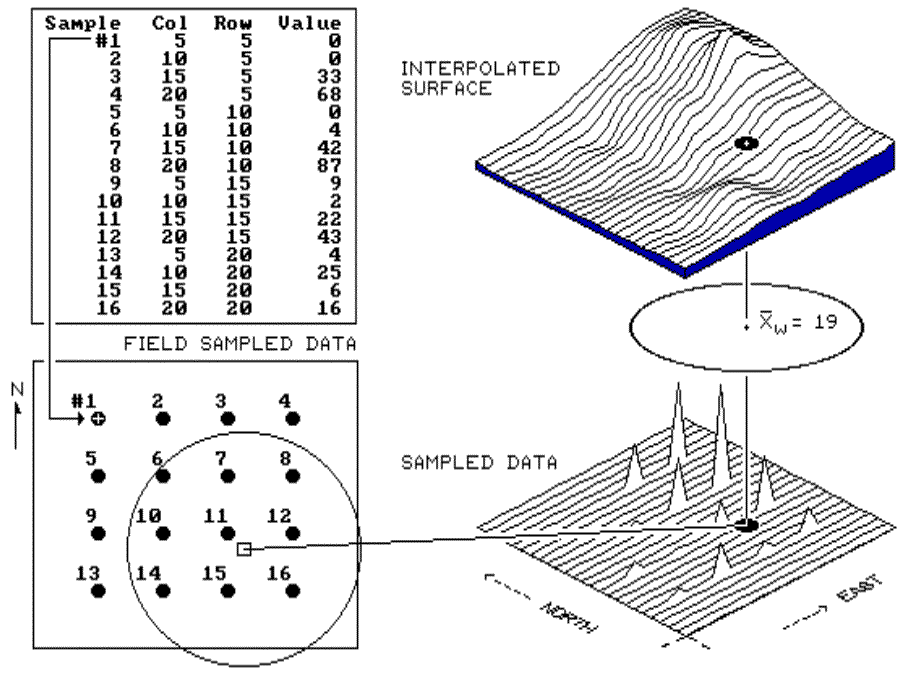Fig. 2.3. Inverse distance interpolation.

Inverse distance is an easy one to conceptualize (see fig. 2.3).  It estimates the value for a location as an average of the data values within its vicinity.  The average is weighted in a manner that decreases the influence of the surrounding sample values as the distance increases.  In figure 2.3, the estimate of 19 is the "inverse distance-squared (1/d2) weighted average" of the nine samples in the window.  Sample #11 (the closest) influences the average a great deal more than sample #6 (the farthest away).   Because this is a static averaging method, the estimated values can never exceed the range of values in the original field data.  Also, inverse distance tends to "pull-down peaks and pull-up valleys" in the data.  The technique is best suited for data sets with random samples that are relatively independent of their surrounding locations (i.e., minimal regional trend).

The right portion of figure 2.3 contains three-dimensional (3-D) plots of the point sample data and the inverse distance-squared surface generated.  The estimated value in the example can be conceptualized as "sitting on the surface," 19 units above the base (zero).  Note that the surface generated by the inverse distance technique is sensitive to sampled locations and tends to put bumps around the sampled areas.).

The inverse distance interpolation technique is fairly straight forward—distance weighted average of nearby sample points.  Kriging and minimum curvature techniques give us two more spatial interpolation tools to explore before we can consider which is the best technique to determine residual analysis.

Obliquely speaking, the Kriging interpolation technique uses "regional variable theory based on an underlying linear variogram."  Without immersion into its theory, it is enough to know that there is a lot of math behind the technique.  In effect, it develops a "custom" window based on the spacing and directional trends it detects in the sample data.   The reach, or size of the window, is determined by intersample correlation.   The summary technique weights the data values, aligning them along the trend's direction more than values opposing the trend.  This trend-weighted moving average can result in estimated values that exceed the actual range of the sampled data.   Also, there can be unexpected results in large areas without data values.  The technique is most appropriate for systematically sampled data exhibiting discernible trends.  The center portion of figure 2.4 depicts the Kriging surface of the sample data described above.  In general, it appears somewhat smoother than the inverse distance plot (left portion) discussed in the previous section.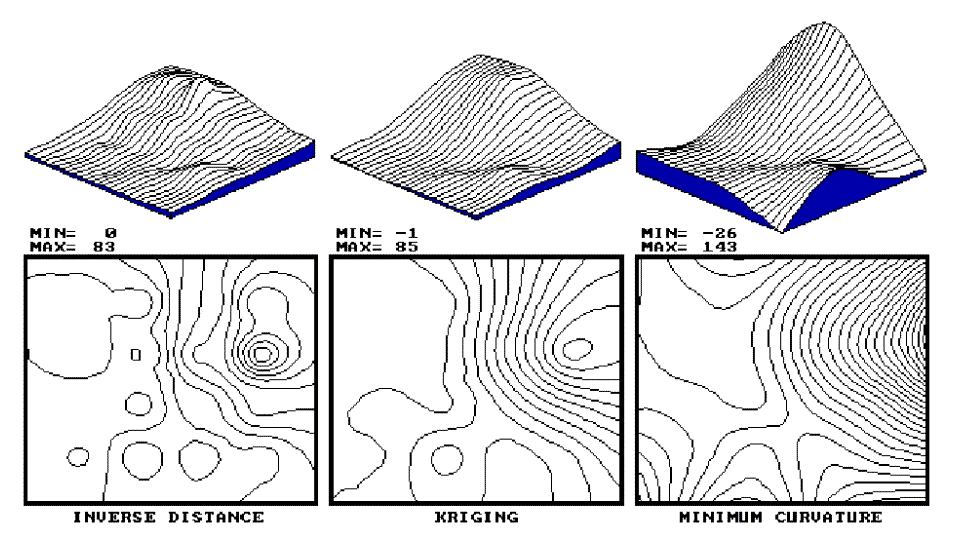Fig. 2.4. Results of Inverse Distance, Kriging vs. Minimum Curvature interpolation.

Another technique, minimum curvature (mincurve), first calculates a set of initial estimates for all map locations based on localized trends in the sampled data, then repeatedly applies a smoothing equation to the surface.  The smoothing continues until successive changes at each map location are less than a specified "maximum absolute deviation," or a maximum number of iterations has been reached.  In practice, the process is done on a coarse map grid and repeated for finer and finer grid spacing until the desired grid spacing and smoothness are reached.  As with Kriging, the estimated values often exceed the range of the original data values and things can go berserk in areas without sample values.

The right portion of figure 2.4 contains the plots for the minimum curvature technique.   Note that it is the smoothest of the three plots and displays a strong edge effect along the border areas that do not contain samples.  This technique is appropriate for systematically sampled data and requires samples close to the border.

By now you are likely to concede that spatial interpolation is a bit overwhelming and it might seem a bit "fishy."  The three plots show radically different renderings for the same set of data.  The actual point sampled data had a range from 0 through 87, but none of the interpolated surfaces matches the actual range.  So, how good are they?  Which rendering is best?

The only thing certain is that there isn’t a single best method that covers all situations.  Some rules of thumb and general considerations have been discussed, but the only good way to determine the "best" map is to empirically verify the results.  This involves generating several maps then testing the results against known measurements, aptly termed a test set.  As in horseshoes and bocci, the closest one wins. Residual analysis summarizes the differences between map estimates and actual measurements.

Figure 2.5 displays a test set containing 16 random samples (#17-32) from the data used in the last two sections.  The "Actual" column lists the measured values for the test locations identified by the "Col," "Row" coordinates.   The difference between these values and those predicted by various interpolation techniques form the residuals shown in parentheses.  The "Average" column compares the whole field arithmetic mean of 23 (guess 23 everywhere) for each test location.  For example, the first sample (#17) guesses 23 but was actually 0; therefore, it’s off by 23.  Note the sign of the residual indicates whether the guesses are too high (positive) or too low (negative).  If it were a perfect data world, you would expect the "Sum of the Residuals" to be 0 (just as many high as low misses, in other words, balanced).  However, in this case there is a net residual of -17 (indicating a bias to underestimate).  The "Average Unsigned Residual" is simply the average magnitude of mistakes, regardless whether more or less than.  For the "Average," expect errors of about 22.8 each time you guess.  The "Normalized Residual Index" is simply the ratio of the "Average Unsigned Residual" to the "Test Set Average."  An index of .81 indicates that the "Average" technique isn’t very good.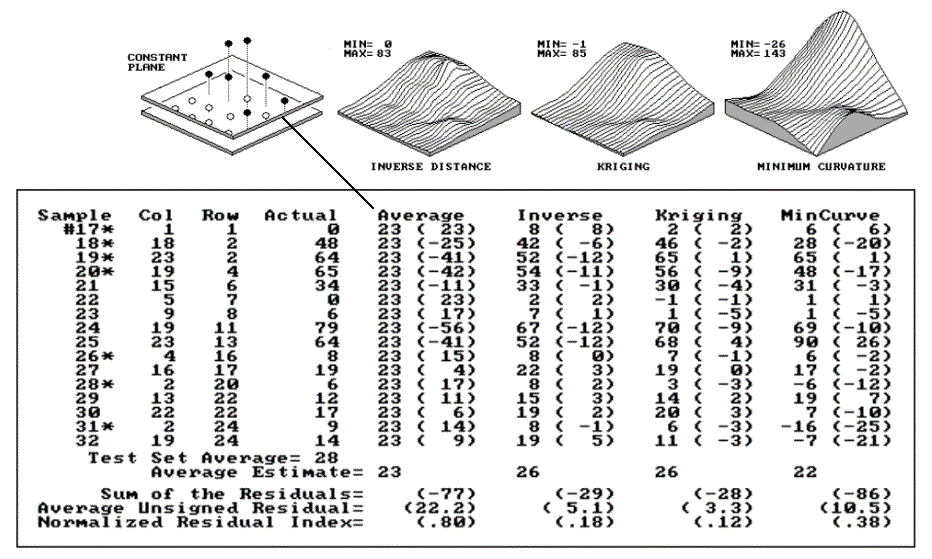Fig. 2.5. Residual analysis table for Average, IDW, Kriging and MinCurve estimates.

Now take a look at the residual analysis for the other techniques.  All three techniques are considerably better than the whole field average (indices of .18, .12 and .38, compared to a whopping .81), with the Kriging technique best, having a 3.3 "Average Unsigned Residual" and a tendency to underestimate (a "Sum of the Residuals" of -28).  The inverse technique is a close second with an index of .18 and a nearly identical underestimate bias (-29).  The mincurve technique seems out of the running.  However, we haven’t considered other potential effects.   The asterisks identify test measurements outside the set of samples used for interpolation.

Is there a significant difference between the various techniques in their estimates for the two different populations? (Get out your calculator.)  I wonder if the conclusions change if the test and interpolation samples are swapped and another residual analysis performed?  What if different sampling designs were tried?  What would be the effect of different interpolation parameters (window reach, number of samples and data balancing)?  Residual analysis gives us a place to start looking for answers.

To summarize, we have developed a procedure for checking the reliability of an interpolated map, such as a map of soil nitrogen derived from a set of soil samples.   It suggests holding back some of the field samples to "empirically verify" the estimated values at the sampled locations.  If you don’t, then you are simply accepting the map as perfect.  The difference between an interpolated "guess" (prediction) and "what is" (measurement) is a residual.   A table of all the residuals for a test set is analyzed to determine the overall accuracy of an interpolated map.  The residual analysis determines that the Kriging interpolation procedure has the closest predictions to the test set measurements.  Its predictions, on average, are only 3.3 units off, while the other techniques were considerably more (5.1 and 10.5).  The residual table identifies the best interpolated map and just how good it is, but it fails to identify where in the field the map is likely predicting too high or too low.  That’s the role of an error map.

Note: See appendix A, part 2, "Comparison of Interpolated and Extrapolated Sets," for a discussion on interpolation and extrapolation.

Figure 2.6 shows both a 3-D and a 2-D map of error for the Kriging technique, derived by interpolating its residuals.  The posted values on the 3-D surface locate the residual values used to derive the map of error.  For example, the value 1 posted for the far corner identifies the residual for test sample #19 at column 23 and row 2; the 4 to its right identifies the residual for test sample #25 at column 23 and row 13 (see fig. 2.5).  The dark lines in both plots locate the zero residual contour—right on target.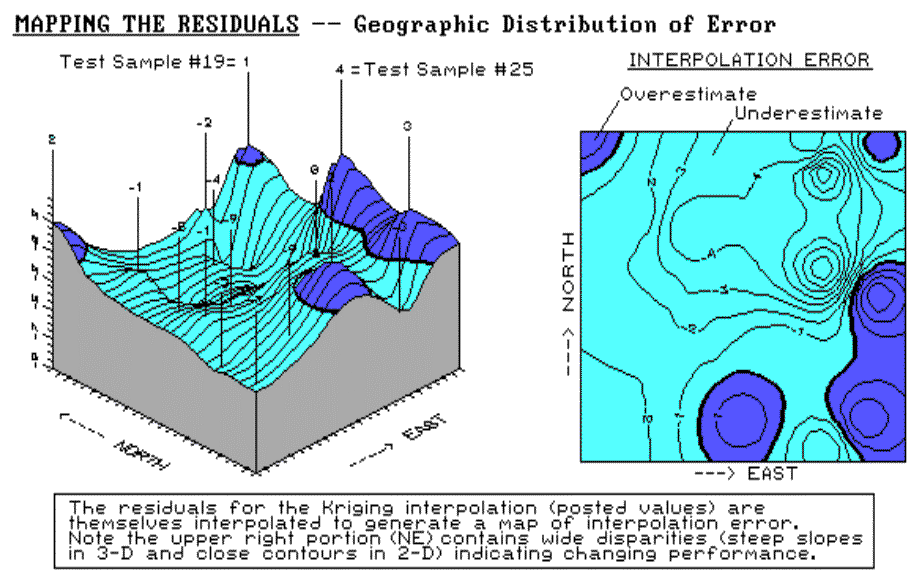Fig. 2.6. Three- and two-dimensional error maps.

It appears that the Kriging overestimates occur along the right (east), bottom (south) and the upper-left (northwest) corner.  Underestimates occur toward the center of the field.  The upper-right portion (northeast) of the error map is particularly interesting.  The two -9 "holes" for test samples #20 and #24 quickly rise to the 1 and 4 "peaks" for samples #19 and #25.  These wide disparities within close proximity of each other (steep slopes in 3-D and tight contour lines in 2-D) indicate areas of wildly differing interpolation performance.  When developing a prescription for this area, the added information on interpolation error might prove extremely helpful.  It certainly takes us beyond simply "pretty maps" toward the mapped data we need in precision farming.

Note: See appendix A, part 2, "Normalized Map Comparisons," for discussion on normalizing maps of error.  Also, see "Visually Comparing Normalized Residual Maps" for a visual comparison of the error maps for the average, nearest neighbors, Kriging and minimum curvature interpolated surfaces.

The reliability of any map based on point data depends on

• the existence of spatial dependency within the data,
• the sampling design employed and
• the interpolation algorithm applied.

So far we have discussed sampling designs and interpolation algorithms.  Now it’s time to turn our attention to the general concept of spatial dependency.

Spatial dependency within a data set simply means that "what happens at one location depends on what is happening around it" (formally termed positive spatial autocorrelation).   This simple concept is the foundation for all spatial interpolation.

The Geary Index (see the "Warning" box) calculates the squared difference between neighboring sample values, then compares their summary to the overall variance for the entire data set.  If the neighboring variance is a lot less than the overall, then considerable dependency is indicated.

The Moran Index is similar; however, it uses the products of neighboring values instead of the differences.  Another approach plots a variogram showing the similarity among locations as a function of the distance between sample points.  Although the calculations themselves vary and arguments abound about the best approach, all of them are reporting the degree of similarity among neighboring point samples.  If there is a lot, then you can generate maps from the data; if there isn’t much, then you are wasting your time.  A "pretty map" can be generated regardless of the degree of spatial dependency, but if dependency is minimal, the map is just colorful gibberish; don’t bet the farm on it.

 Warning The following ugly equation is suitable for detailed viewing by only the technically astute (techy-types): c= [ (n-1) SUMi SUMj wij (xi - xj)2 ] / [ 2 * SUMi SUMj wij * SUMi (xi - a)2 ]          (Eq. T2.1) where x = sample value a = mean of x values n = number of samples wij = 1 if i,j adjacent (closest pair), else 0 The rest of us are suitably impressed and recognize that such an index can help to evaluate an interpolated map’s reliability.  If c = 1, then neighboring samples vary as much as the overall data set and no spatial dependency exists.  Any map generated from the data is pure, dense gibberish.   If c < 1, then the neighboring samples are more similar (increasing positive spatial autocorrelation) and interpolation is justified.  Not that all of us understand the Geary equation, but as intelligent consumers of precision farming products we are putting the vendors on notice that such tests of map reliability should accompany all "pretty maps" (as well as really neat graphics and vibrant colors).

If you have survived the previous discussions about the basics of "traditional" statistics, let’s turn our attention to spatial statistics.   Its basic concept involves spatial autocorrelation, referring to the degree of similarity among neighboring points (e.g., soil nutrient samples).  If they exhibit a lot similarity, or spatial dependence, they ought to derive a good map.   If they are spatially independent, then expect a map of pure, dense gibberish.   So how can we measure whether "what happens at one location depends on what is happening around it?"

Common sense leads us to believe more similarity exists among the neighboring soil samples (lines in the left side of fig. 2.7) than among sample points farther away.   The Geary and Moran Indices consider the closest neighbors.  They compute the differences in the values between each sample point and its closest neighbor.  If the differences in neighboring values are a lot smaller than the overall variation among all samples, then a high degree of positive spatial dependency is indicated.  If they are about the same or if the neighbors variation is larger (a rare "checkerboard-like" condition), then the assumption of spatial dependence fails.  If the dependency test fails, it means the soil nutrient map is just colorful gibberish.  You should ask to see the Geary and Moran Indices before you accept any soil nutrient map.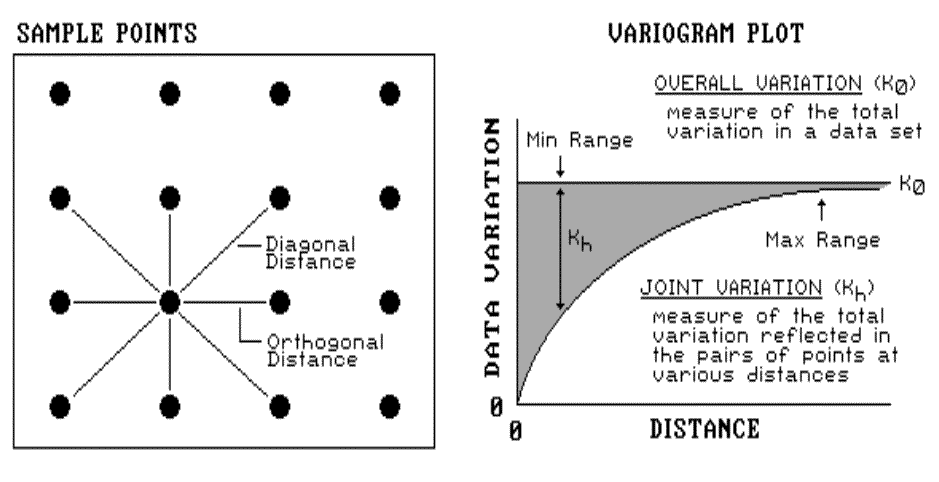Fig. 2.7. Variogram plot.

These indices, however, are limited as they merely assess the closest neighbor, regardless of its distance.  That’s where a variogram comes in.  It is a plot (neither devious nor spiteful) of the similarity among values based on the distance between them.  Instead of simply testing whether close things are related, it shows how the degree of dependency relates to varying distances between locations.  The origin of the plot at 0,0 is a unique case.   The distance between samples is zero; therefore, the dissimilarity (data variation = 0) is exactly the same as itself.

As the distance between points increases, subsets of the data are scrutinized for their dependency.  The shaded portion in the plot shows how quickly the spatial dependency among points deteriorates with distance.  The maximum range position identifies the distance between points beyond which the data values are considered to be independent of one another.  This tells us that using data values beyond this distance for interpolation actually can mess-up the interpolation.  The minimum range position identifies the smallest distance actually contained in the data set.  If most of the shaded area falls below this distance, it tells you there is insufficient spatial dependency in the data set to warrant interpolation.  If you proceed with the interpolation, a nifty colorful map will be generated, but it’ll be less than worthless.

You should ask to see the variogram plot, as well as the Geary and Moran Indices, before you bet the farm on any soil nutrient map.  If they are unavailable, then the map is just "a pig in the poke."

Note: More information on spatial dependency is contained in topic 3, "Assessing Spatial Dependency," and appendix A, part 2, "More on Assessing Spatial Dependency."

Zones and Surfaces

Site-specific farming involves "carving" a field into smaller pieces that better represent the unique conditions and patterns occurring in the field.  Two fundamental approaches are used: management zones and map surfaces (see fig. 2.8). Management zones use a farmer’s knowledge, air photos, terrain features, yield maps or other factors to identify discrete areas considered homogeneous.  Sampling, analysis and management decisions are undertaken for each distinct zone—as if they were separate, mini-fields.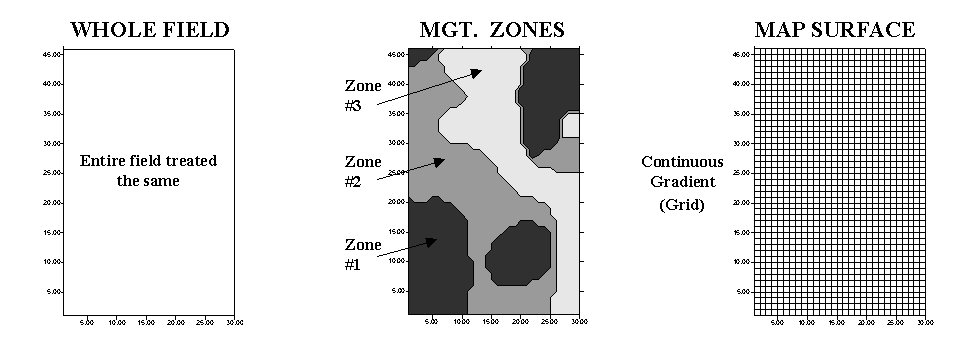Fig. 2.8. Comparison of approaches to subdivide the field.

Map surfaces, on the other hand, treat a field as a continuous surface by partitioning it into thousands of grid cells that track gradual transitions throughout the field.  The resulting grid spaces represent tiny snippets of the field, and information is assigned to each to track the pattern of variation.

Both approaches have their advantages, and disadvantages—management zones are intuitive, require minimal data collection and are less expensive to implement.   Map surfaces, on the other hand, are not constrained by artificially abrupt boundaries, therefore they better describe field variability and have greater analysis capabilities.  "Like all things GIS," an understanding of the nature of the data and the assumptions underlying the approaches provide insight into their differences.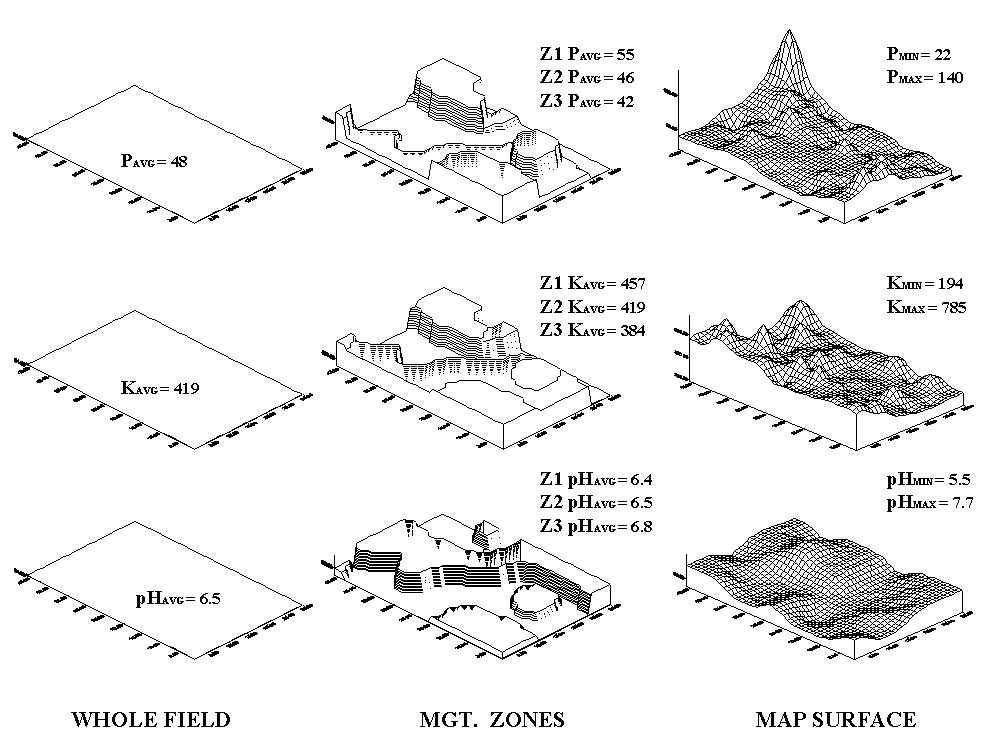Fig. 2.9. Comparison of soil data maps generated by the different approaches.

Consider the maps of P, K and pH shown in figure 2.9.  The "Whole Field" representations are characterized as three horizontal planes "floating" at their average values—the same throughout the entire field.  The "Management Zones" approach depicts a "plateau" for each of the three zones determined by their averages—the same throughout each zone.  Note that Zone 3 shows lower P and K (42 and 384) but higher pH (6.8) than the whole field averages (48, 419 and 6.5, respectively).

Now consider the "Map Surfaces" that were interpolated from the same soil samples used by the other two approaches.  In a sense, the approach maps the variance in the data instead of assigning its average everywhere.  The maps characterize the field as a gradient—constantly varying.  Note the large phosphorous peak in the NE portion of the field (maximum = 140) and the low values in the SE (minimum = 22).  The other surfaces also locate areas that are well above and below the "Whole Field" and "Management Zones" averages.

 Table 2.1. Statistical summary of approaches to subdivide the field.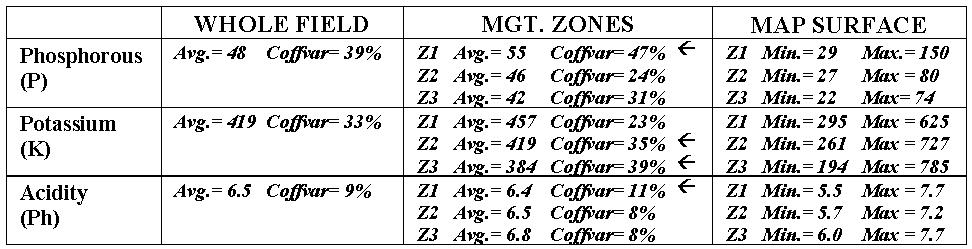The differences among the approaches also show up in statistical summaries (see table 2.1).  Recall that the coefficient of variation (Coffvar) is a frequently used measure that indicates the amount of variation in a set of data—with greater numbers indicating more variation.  The "Whole Field" Coffvar’s tell us that, throughout the field, there is a fair amount of variation in the P and K values (39% and 33%), but not much for pH (9%).  Since the "Management Zones" approach breaks the field into smaller units that are assumed to be more homogenous, it is expected that the Coffvar’s for the zones would be less than those of the "Whole Field."

In most cases they are, but the exceptions (identified by arrows in the table) are interesting.  They identify zones where the subdividing isn’t very good and the averages of the zones are misleading.  Note that the data ranges (minimum to maximum value as depicted by the "Map Surfaces") are very large for these zones.  For example, zone 1 with a coffvar of 47% has phosphorous values ranging from 29 to 150 (a five-fold difference).  Similarly, the pH range (5.5 to 7.7) for the zone is fairly large.  The real problem arises when non-typical conditions align in space, such as the NE corner in zone 1.  As both the "Whole Field" and "Management Zones" approaches assume the typical response (average) is everywhere, they miss the combined effects of subtle (and not so subtle) differences from the averages contained in the "Map Surfaces."  The result could be significant differences in a prescription for variable rate application of fertilizer.  While "Management Zones" is a start toward precision farming and site-specific management, it can fall a fair distance short—it’s all in the data and its spatial coincidence.

Note: See appendix A, part 2, "More on Zones and Surfaces" and "Last Word on Zones and Surfaces" for extended discussion of the differences between Management Zones and Map Surfaces approaches to subdividing a field.  Also, an Excel worksheet supporting these discussions is available online at appendix A, part 2, "Excel Worksheet Investigating Zones and Surfaces."